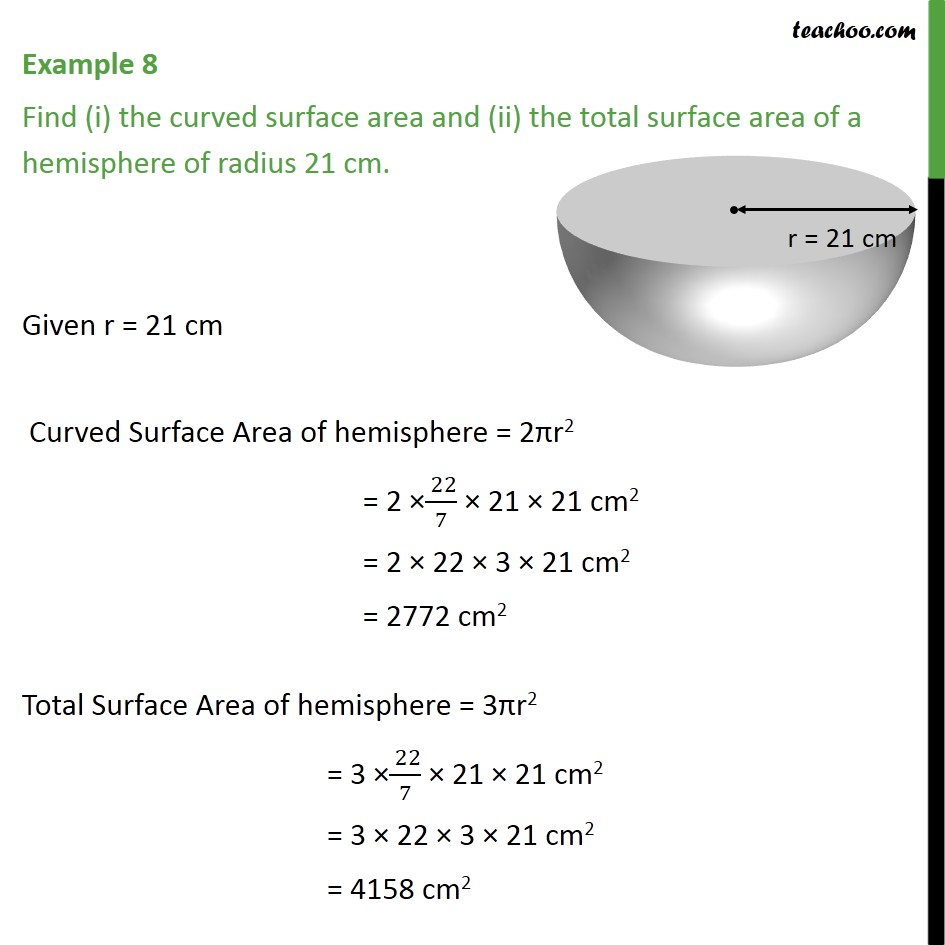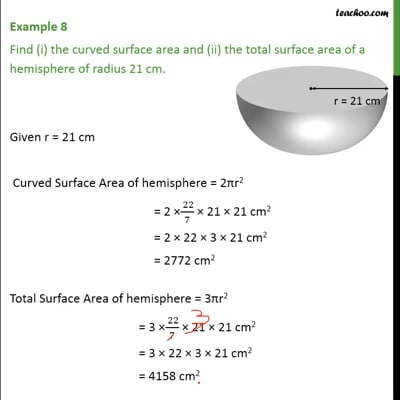Examples

Chapter 13 Class 9 Surface Areas and Volumes
Serial order wiseThis video is only available for Teachoo black users

Maths Crash Course - Live lectures + all videos + Real time Doubt solving!

### Transcript

Example 8 Find (i) the curved surface area and (ii) the total surface area of a hemisphere of radius 21 cm. Given r = 21 cm Curved Surface Area of hemisphere = 2πr2 = 2 × (22)/7 × 21 × 21 cm2 = 2 × 22 × 3 × 21 cm2 = 2772 cm2 Total Surface Area of hemisphere = 3πr2 = 3 ×( 22)/7 × 21 × 21 cm2 = 3 × 22 × 3 × 21 cm2 = 4158 cm2